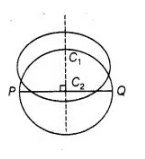# If a number of circles pass through the end

Question:

If a number of circles pass through the end points P and Q of a line segment PQ, then their centres lie on the perpendicular bisector of PQ.

Solution:True

We draw two circle with centres $C_{1}$ and $C_{2}$ passing through the end points $P$ and $Q$ of a line segment $P Q$. We know, that perpendicular bisectors of a chord of a circle always passes through the centre of circle.

Thus, perpendicular bisector of $P Q$ passes through $C_{1}$ and $C_{2}$. Similarly, all the circle passing through $P Q$ will have their centre on perpendiculars bisectors of $P Q$.